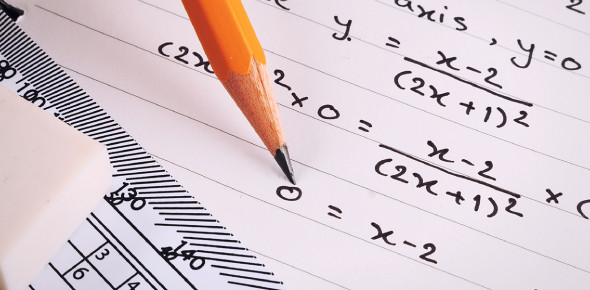# Ultimate Math Exam Trivia: Quiz!

35 Questions | Total Attempts: 106SettingsCreate your own Quiz.

• 1.
A complex number has:
• A.

Real part

• B.

Imaginary part

• C.

Both

• D.

None of them

• 2.
Graphical representation of the complex numbers is called:
• A.

Argands diagram

• B.

Polar form

• C.

Non polar form

• D.

None of these

• 3.
Sin2x is:
• A.

2sinxcosx

• B.

Sin2xcos2x

• C.

Both

• D.

None

• 4.
Derivative of cos(x)
• A.

-sin(x)

• B.

Sin(x)

• C.

Cot(x)

• D.

None of these

• 5.
Derivative of ln(x)
• A.

1/x

• B.

Ln(x)

• C.

1

• D.

Ex

• 6.
A derivative of tan(x)
• A.

Sec2(x)

• B.

Cot(x)

• C.

Sec(x)

• D.

Cosec2(x)

• 7.
A derivative of eis
• A.

1

• B.

0

• C.

Ex

• D.

None of these

• 8.
A derivative of 2x3 is
• A.

6x

• B.

6x2

• C.

12x

• D.

None of these

• 9.
A derivative of cos x is
• A.

-sin x

• B.

Sin x

• C.

Cosx sinx

• D.

None of these

• 10.
A derivative of sec x is
• A.

-sec x tan x

• B.

-cosec2x

• C.

-cos x

• D.

Cot2x

• 11.
A matrix is said to be _______ if the number of rows is not equal to the number of columns of a matrix.
• A.

Rectangular

• B.

Square

• C.

Diagonal

• D.

None of these

• 12.
A square matrix A is said to be ______ if |A| is equal to 0.
• A.

Singular matrix

• B.

Non-singular matrix

• C.

Triangular matrix

• D.

None of these

• 13.
A square matrix A is said to be ______ if |A| is not equal to 0.
• A.

Non singular matrix

• B.

Singular matrix

• C.

Triangular

• D.

None of these

• 14.
A square matrix A is equal to [aij] is said to be ______ if [aij] = [aji]
• A.

Symmetric

• B.

Asymmetric

• C.

Conjugate

• D.

None of these

• 15.
State the reflexive property.
• A.

A = A

• B.

If A=B then B=A

• C.

If A=B ,B=C then A=C

• D.

Option 4

• 16.
AA-1 = ?
• A.

I

• B.

1

• C.

0

• D.

None of these

• 17.
A matrix is said to be ________ if all the non-diagonal elements are 0.
• A.

Diagonal

• B.

Square

• C.

Column

• D.

Circular

• 18.
A derivative of cot x
• A.

-cosec2 x

• B.

Sec2 x

• C.

-tan x

• D.

Cos2 x

• 19.
∫ sinx dx
• A.

- cos x

• B.

Cos x

• C.

Sec x

• D.

-cosec x

• 20.
∫ cosx dx
• A.

Sin x

• B.

-sin x

• C.

-cosec x

• D.

Sec x

• 21.
∫ cosec2x dx
• A.

-cotx

• B.

-tanx

• C.

Cotx

• D.

Tanx

• 22.
Sin2x + cos2x = ?
• A.

1

• B.

Sin2x

• C.

Cos2x

• D.

-1

• 23.
An ___________ set of mn numbers arranged in a rectangular array of m rows and n columns and enclosed by a pair of brackets is called a matrix.
• A.

Ordered

• B.

Unordered

• C.

Evenly ordered

• D.

None of them

• 24.
Which of these is not a matrix type?
• A.

Rounded matrix

• B.

Row

• C.

Column

• D.

None of these

• 25.
The determinant of a matrix is mentioned as?
• A.

Mod

• B.

Hash

• C.

Tilde

• D.

None of these

Related TopicsBack to top
×

Wait!
Here's an interesting quiz for you.